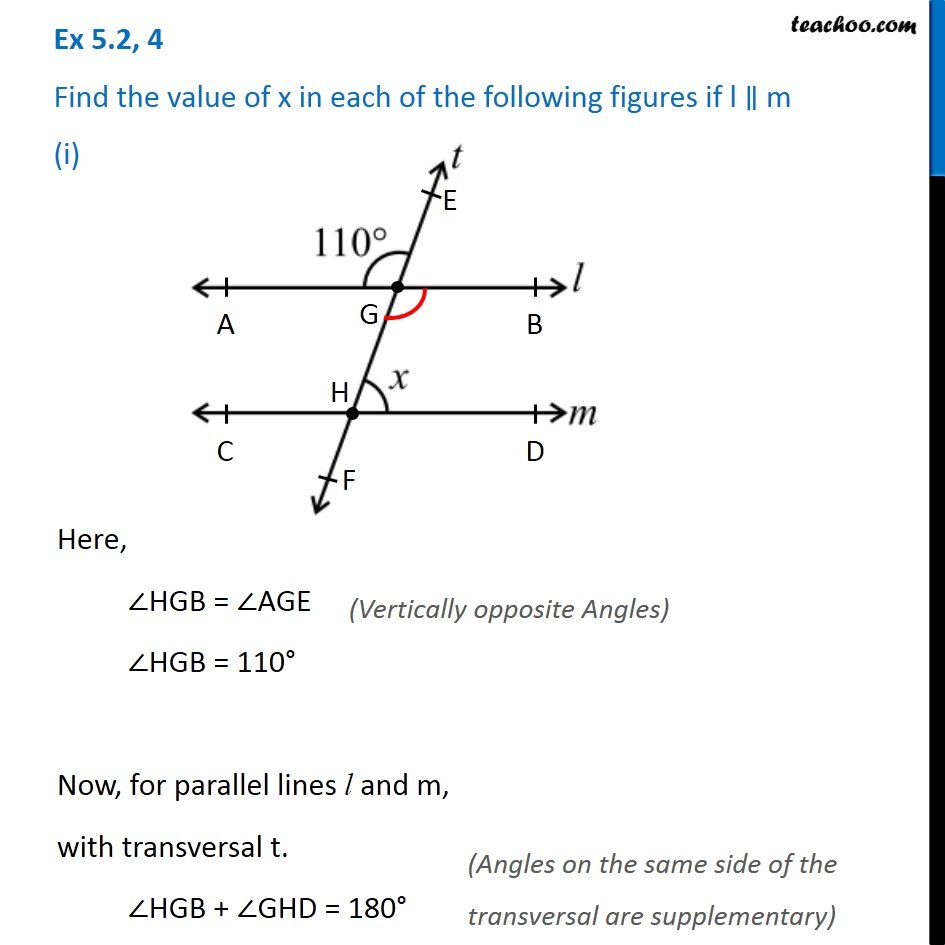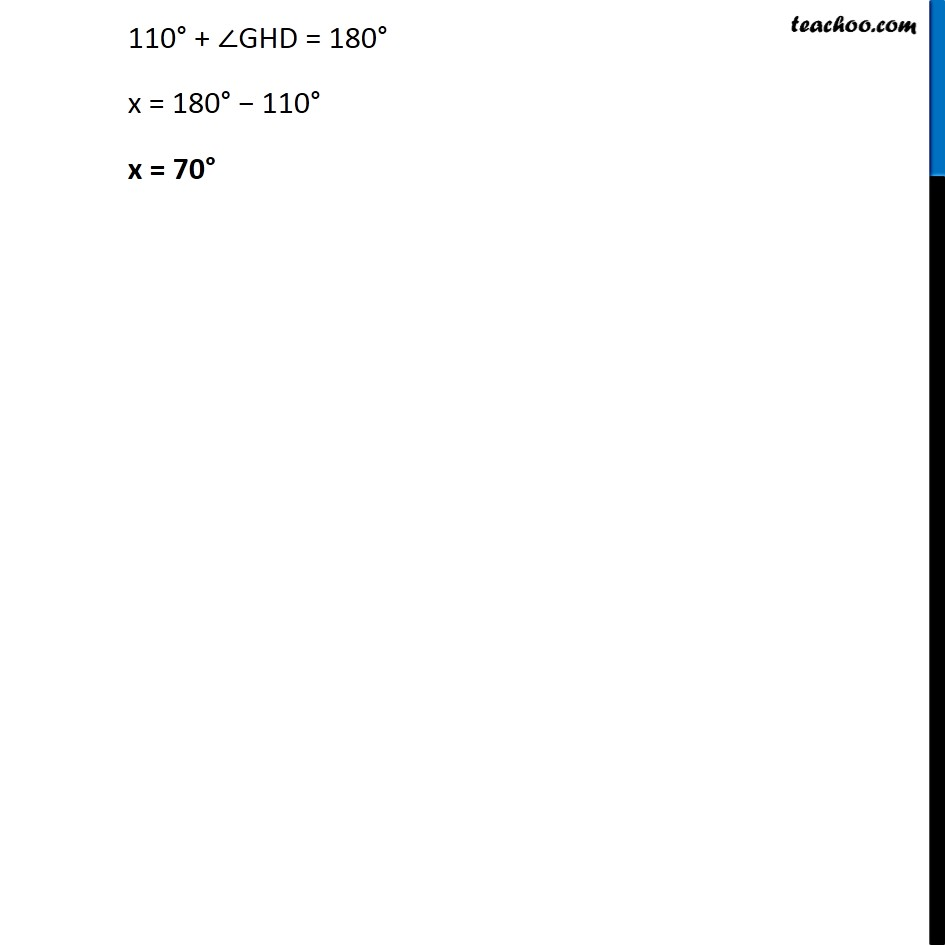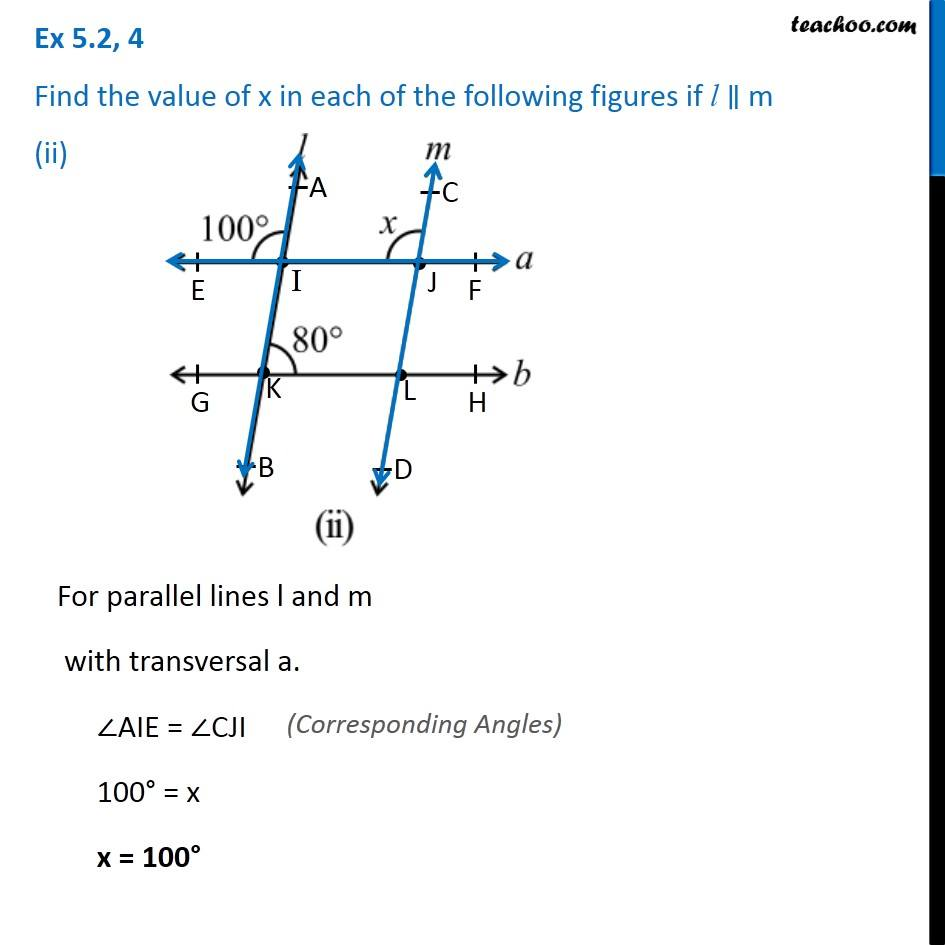Ex 5.2

Chapter 5 Class 7 Lines and Angles
Serial order wiseLearn in your speed, with individual attention - Teachoo Maths 1-on-1 Class

### Transcript

Ex 5.2, 4 Find the value of x in each of the following figures if l ∥ m (i)Here, ∠HGB = ∠AGE ∠HGB = 110° Now, for parallel lines l and m, with transversal t. ∠HGB + ∠GHD = 180° 110° + ∠GHD = 180° x = 180° − 110° x = 70° Ex 5.2, 4 Find the value of x in each of the following figures if l ∥ m (ii)For parallel lines l and m with transversal a. ∠AIE = ∠CJI 100° = x x = 100°